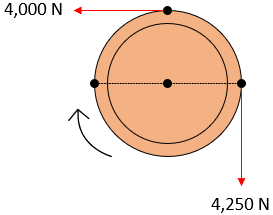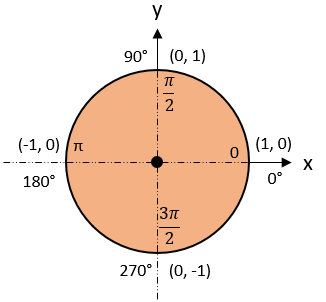## Pulley + Belt

In the figure shown, a pulley is driven by a belt. What minimum coefficient of friction will prevent slipping between pulley and belt? Ignore centrifugal effects.Hint
Belt Friction:
$$F_1=F_2e^{\mu \theta}$$$where $$F_1$$ is the applied force in the direction of impending motion, $$F_2$$ is the applied force resisting impending motion, $$\mu$$ is the static coefficient of friction, and $$\theta$$ is the total angle of contact between surfaces in radians. Hint 2 Unit Circle:Belt Friction: $$F_1=F_2e^{\mu \theta}$$$
where $$F_1$$ is the applied force in the direction of impending motion, $$F_2$$ is the applied force resisting impending motion, $$\mu$$ is the static coefficient of friction, and $$\theta$$ is the total angle of contact between surfaces in radians. Recall a unit circle to determine $$\theta$$ :Therefore,
$$4,250N=(4,000N)e^{\mu \pi/2}$$$Solving for the coefficient of friction: $$ln(\frac{4,250}{4,000})=ln(e^{\mu \pi/2})=\mu \frac{\pi }{2}$$$
$$\mu=\frac{2}{\pi}\cdot ln(\frac{4,250}{4,000})=\frac{2}{\pi} \cdot 0.06=0.04$$\$
0.04